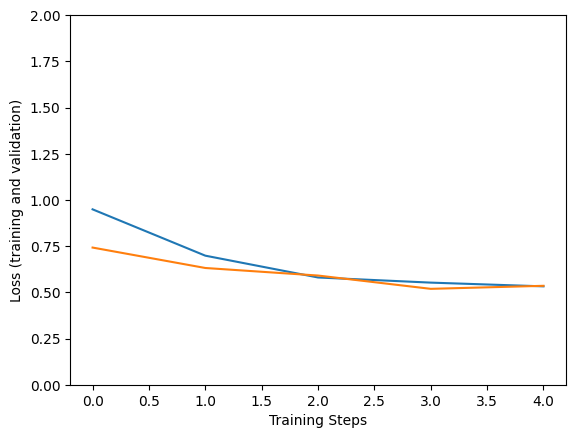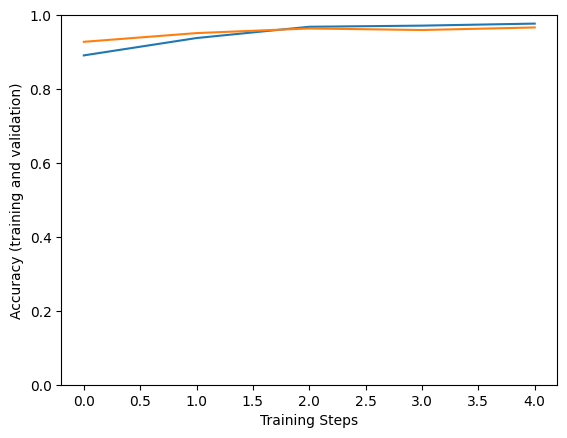# Retraining an Image Classifier

## Introduction

Image classification models have millions of parameters. Training them from scratch requires a lot of labeled training data and a lot of computing power. Transfer learning is a technique that shortcuts much of this by taking a piece of a model that has already been trained on a related task and reusing it in a new model.

This Colab demonstrates how to build a Keras model for classifying five species of flowers by using a pre-trained TF2 SavedModel from TensorFlow Hub for image feature extraction, trained on the much larger and more general ImageNet dataset. Optionally, the feature extractor can be trained ("fine-tuned") alongside the newly added classifier.

### Looking for a tool instead?

This is a TensorFlow coding tutorial. If you want a tool that just builds the TensorFlow or TFLite model for, take a look at the make_image_classifier command-line tool that gets installed by the PIP package `tensorflow-hub[make_image_classifier]`, or at this TFLite colab.

## Setup

``````import itertools
import os

import matplotlib.pylab as plt
import numpy as np

import tensorflow as tf
import tensorflow_hub as hub

print("TF version:", tf.__version__)
print("Hub version:", hub.__version__)
print("GPU is", "available" if tf.config.list_physical_devices('GPU') else "NOT AVAILABLE")
``````
```TF version: 2.6.0
Hub version: 0.12.0
GPU is available
```

## Select the TF2 SavedModel module to use

For starters, use https://hub.tensorflow.google.cn/google/imagenet/mobilenet_v2_100_224/feature_vector/4. The same URL can be used in code to identify the SavedModel and in your browser to show its documentation. (Note that models in TF1 Hub format won't work here.)

You can find more TF2 models that generate image feature vectors here.

There are multiple possible models to try. All you need to do is select a different one on the cell below and follow up with the notebook.

``````model_name = "efficientnetv2-xl-21k" # @param ['efficientnetv2-s', 'efficientnetv2-m', 'efficientnetv2-l', 'efficientnetv2-s-21k', 'efficientnetv2-m-21k', 'efficientnetv2-l-21k', 'efficientnetv2-xl-21k', 'efficientnetv2-b0-21k', 'efficientnetv2-b1-21k', 'efficientnetv2-b2-21k', 'efficientnetv2-b3-21k', 'efficientnetv2-s-21k-ft1k', 'efficientnetv2-m-21k-ft1k', 'efficientnetv2-l-21k-ft1k', 'efficientnetv2-xl-21k-ft1k', 'efficientnetv2-b0-21k-ft1k', 'efficientnetv2-b1-21k-ft1k', 'efficientnetv2-b2-21k-ft1k', 'efficientnetv2-b3-21k-ft1k', 'efficientnetv2-b0', 'efficientnetv2-b1', 'efficientnetv2-b2', 'efficientnetv2-b3', 'efficientnet_b0', 'efficientnet_b1', 'efficientnet_b2', 'efficientnet_b3', 'efficientnet_b4', 'efficientnet_b5', 'efficientnet_b6', 'efficientnet_b7', 'bit_s-r50x1', 'inception_v3', 'inception_resnet_v2', 'resnet_v1_50', 'resnet_v1_101', 'resnet_v1_152', 'resnet_v2_50', 'resnet_v2_101', 'resnet_v2_152', 'nasnet_large', 'nasnet_mobile', 'pnasnet_large', 'mobilenet_v2_100_224', 'mobilenet_v2_130_224', 'mobilenet_v2_140_224', 'mobilenet_v3_small_100_224', 'mobilenet_v3_small_075_224', 'mobilenet_v3_large_100_224', 'mobilenet_v3_large_075_224']

model_handle_map = {
}

model_image_size_map = {
"efficientnetv2-s": 384,
"efficientnetv2-m": 480,
"efficientnetv2-l": 480,
"efficientnetv2-b0": 224,
"efficientnetv2-b1": 240,
"efficientnetv2-b2": 260,
"efficientnetv2-b3": 300,
"efficientnetv2-s-21k": 384,
"efficientnetv2-m-21k": 480,
"efficientnetv2-l-21k": 480,
"efficientnetv2-xl-21k": 512,
"efficientnetv2-b0-21k": 224,
"efficientnetv2-b1-21k": 240,
"efficientnetv2-b2-21k": 260,
"efficientnetv2-b3-21k": 300,
"efficientnetv2-s-21k-ft1k": 384,
"efficientnetv2-m-21k-ft1k": 480,
"efficientnetv2-l-21k-ft1k": 480,
"efficientnetv2-xl-21k-ft1k": 512,
"efficientnetv2-b0-21k-ft1k": 224,
"efficientnetv2-b1-21k-ft1k": 240,
"efficientnetv2-b2-21k-ft1k": 260,
"efficientnetv2-b3-21k-ft1k": 300,
"efficientnet_b0": 224,
"efficientnet_b1": 240,
"efficientnet_b2": 260,
"efficientnet_b3": 300,
"efficientnet_b4": 380,
"efficientnet_b5": 456,
"efficientnet_b6": 528,
"efficientnet_b7": 600,
"inception_v3": 299,
"inception_resnet_v2": 299,
"nasnet_large": 331,
"pnasnet_large": 331,
}

model_handle = model_handle_map.get(model_name)
pixels = model_image_size_map.get(model_name, 224)

print(f"Selected model: {model_name} : {model_handle}")

IMAGE_SIZE = (pixels, pixels)
print(f"Input size {IMAGE_SIZE}")

BATCH_SIZE = 16
``````
```Selected model: efficientnetv2-xl-21k : https://hub.tensorflow.google.cn/google/imagenet/efficientnet_v2_imagenet21k_xl/feature_vector/2
Input size (512, 512)
```

## Set up the Flowers dataset

Inputs are suitably resized for the selected module. Dataset augmentation (i.e., random distortions of an image each time it is read) improves training, esp. when fine-tuning.

``````data_dir = tf.keras.utils.get_file(
'flower_photos',
untar=True)
``````
```Downloading data from https://storage.googleapis.com/download.tensorflow.org/example_images/flower_photos.tgz
228818944/228813984 [==============================] - 7s 0us/step
228827136/228813984 [==============================] - 7s 0us/step
```

```Found 3670 files belonging to 5 classes.
Using 2936 files for training.
Found 3670 files belonging to 5 classes.
Using 734 files for validation.
```

## Defining the model

All it takes is to put a linear classifier on top of the `feature_extractor_layer` with the Hub module.

For speed, we start out with a non-trainable `feature_extractor_layer`, but you can also enable fine-tuning for greater accuracy.

``````do_fine_tuning = False
``````
``````print("Building model with", model_handle)
model = tf.keras.Sequential([
# Explicitly define the input shape so the model can be properly
tf.keras.layers.InputLayer(input_shape=IMAGE_SIZE + (3,)),
hub.KerasLayer(model_handle, trainable=do_fine_tuning),
tf.keras.layers.Dropout(rate=0.2),
tf.keras.layers.Dense(len(class_names),
kernel_regularizer=tf.keras.regularizers.l2(0.0001))
])
model.build((None,)+IMAGE_SIZE+(3,))
model.summary()
``````
```Building model with https://hub.tensorflow.google.cn/google/imagenet/efficientnet_v2_imagenet21k_xl/feature_vector/2
Model: "sequential_1"
_________________________________________________________________
Layer (type)                 Output Shape              Param #
=================================================================
keras_layer (KerasLayer)     (None, 1280)              207615832
_________________________________________________________________
dropout (Dropout)            (None, 1280)              0
_________________________________________________________________
dense (Dense)                (None, 5)                 6405
=================================================================
Total params: 207,622,237
Trainable params: 6,405
Non-trainable params: 207,615,832
_________________________________________________________________
```

## Training the model

``````model.compile(
optimizer=tf.keras.optimizers.SGD(learning_rate=0.005, momentum=0.9),
loss=tf.keras.losses.CategoricalCrossentropy(from_logits=True, label_smoothing=0.1),
metrics=['accuracy'])
``````
``````steps_per_epoch = train_size // BATCH_SIZE
validation_steps = valid_size // BATCH_SIZE
hist = model.fit(
train_ds,
epochs=5, steps_per_epoch=steps_per_epoch,
validation_data=val_ds,
validation_steps=validation_steps).history
``````
```Epoch 1/5
183/183 [==============================] - 128s 536ms/step - loss: 0.8901 - accuracy: 0.8958 - val_loss: 0.6734 - val_accuracy: 0.9458
Epoch 2/5
183/183 [==============================] - 94s 510ms/step - loss: 0.6460 - accuracy: 0.9442 - val_loss: 0.7162 - val_accuracy: 0.9375
Epoch 3/5
183/183 [==============================] - 93s 507ms/step - loss: 0.6038 - accuracy: 0.9572 - val_loss: 0.5917 - val_accuracy: 0.9625
Epoch 4/5
183/183 [==============================] - 93s 507ms/step - loss: 0.5454 - accuracy: 0.9712 - val_loss: 0.5671 - val_accuracy: 0.9569
Epoch 5/5
183/183 [==============================] - 93s 508ms/step - loss: 0.5296 - accuracy: 0.9767 - val_loss: 0.5607 - val_accuracy: 0.9681
```
``````plt.figure()
plt.ylabel("Loss (training and validation)")
plt.xlabel("Training Steps")
plt.ylim([0,2])
plt.plot(hist["loss"])
plt.plot(hist["val_loss"])

plt.figure()
plt.ylabel("Accuracy (training and validation)")
plt.xlabel("Training Steps")
plt.ylim([0,1])
plt.plot(hist["accuracy"])
plt.plot(hist["val_accuracy"])
``````
```[<matplotlib.lines.Line2D at 0x7f212179b150>]
```Try out the model on an image from the validation data:

``````x, y = next(iter(val_ds))
image = x[0, :, :, :]
true_index = np.argmax(y)
plt.imshow(image)
plt.axis('off')
plt.show()

# Expand the validation image to (1, 224, 224, 3) before predicting the label
prediction_scores = model.predict(np.expand_dims(image, axis=0))
predicted_index = np.argmax(prediction_scores)
print("True label: " + class_names[true_index])
print("Predicted label: " + class_names[predicted_index])
`````````True label: sunflowers
Predicted label: sunflowers
```

Finally, the trained model can be saved for deployment to TF Serving or TFLite (on mobile) as follows.

``````saved_model_path = f"/tmp/saved_flowers_model_{model_name}"
tf.saved_model.save(model, saved_model_path)
``````
```2021-10-16 11:22:37.251425: W tensorflow/python/util/util.cc:348] Sets are not currently considered sequences, but this may change in the future, so consider avoiding using them.
WARNING:absl:Found untraced functions such as restored_function_body, restored_function_body, restored_function_body, restored_function_body, restored_function_body while saving (showing 5 of 3985). These functions will not be directly callable after loading.
INFO:tensorflow:Assets written to: /tmp/saved_flowers_model_efficientnetv2-xl-21k/assets
INFO:tensorflow:Assets written to: /tmp/saved_flowers_model_efficientnetv2-xl-21k/assets
```

## Optional: Deployment to TensorFlow Lite

TensorFlow Lite lets you deploy TensorFlow models to mobile and IoT devices. The code below shows how to convert the trained model to TFLite and apply post-training tools from the TensorFlow Model Optimization Toolkit. Finally, it runs it in the TFLite Interpreter to examine the resulting quality

• Converting without optimization provides the same results as before (up to roundoff error).
• Converting with optimization without any data quantizes the model weights to 8 bits, but inference still uses floating-point computation for the neural network activations. This reduces model size almost by a factor of 4 and improves CPU latency on mobile devices.
• On top, computation of the neural network activations can be quantized to 8-bit integers as well if a small reference dataset is provided to calibrate the quantization range. On a mobile device, this accelerates inference further and makes it possible to run on accelerators like EdgeTPU.

### Optimization settings

```2021-10-16 11:23:45.870533: W tensorflow/compiler/mlir/lite/python/tf_tfl_flatbuffer_helpers.cc:351] Ignored output_format.
2021-10-16 11:23:45.870583: W tensorflow/compiler/mlir/lite/python/tf_tfl_flatbuffer_helpers.cc:354] Ignored drop_control_dependency.
2021-10-16 11:23:45.870589: W tensorflow/compiler/mlir/lite/python/tf_tfl_flatbuffer_helpers.cc:360] Ignored change_concat_input_ranges.
Wrote TFLite model of 826155352 bytes.
```
``````interpreter = tf.lite.Interpreter(model_content=lite_model_content)
# This little helper wraps the TFLite Interpreter as a numpy-to-numpy function.
def lite_model(images):
interpreter.allocate_tensors()
interpreter.set_tensor(interpreter.get_input_details()['index'], images)
interpreter.invoke()
return interpreter.get_tensor(interpreter.get_output_details()['index'])
``````
``````num_eval_examples = 50
eval_dataset = ((image, label)  # TFLite expects batch size 1.
for batch in train_ds
for (image, label) in zip(*batch))
count = 0
count_lite_tf_agree = 0
count_lite_correct = 0
for image, label in eval_dataset:
probs_lite = lite_model(image[None, ...])
probs_tf = model(image[None, ...]).numpy()
y_lite = np.argmax(probs_lite)
y_tf = np.argmax(probs_tf)
y_true = np.argmax(label)
count +=1
if y_lite == y_tf: count_lite_tf_agree += 1
if y_lite == y_true: count_lite_correct += 1
if count >= num_eval_examples: break
print("TFLite model agrees with original model on %d of %d examples (%g%%)." %
(count_lite_tf_agree, count, 100.0 * count_lite_tf_agree / count))
print("TFLite model is accurate on %d of %d examples (%g%%)." %
(count_lite_correct, count, 100.0 * count_lite_correct / count))
``````
```TFLite model agrees with original model on 50 of 50 examples (100%).
TFLite model is accurate on 49 of 50 examples (98%).
```
[]
[]